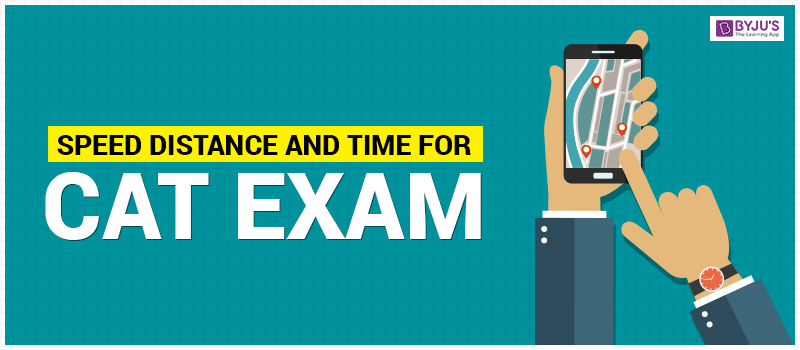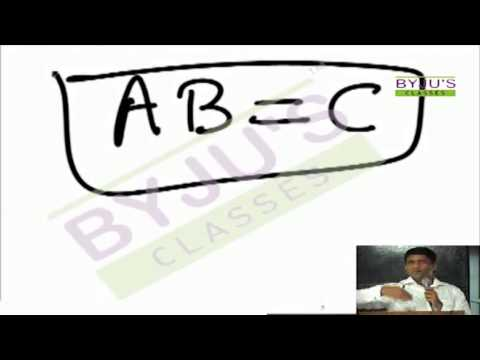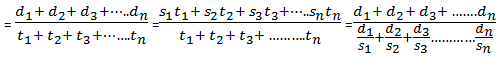# Speed, Distance and TimeIntroduction- Speed Distance Time is one of the most popular and important topics in CAT exam. Around 5% to 10% questions in the exam are asked from Speed Distance Time every year. The concept of this chapter is also used extensively for questions relating different sections such as motion in a straight line, circular motion, boats and streams, races, clocks, etc. Aspirants should try to understand the inter-relationship between the factors speed, distance and time.

## Relationship between Speed, Distance and Time

We know that,

### Distance = Speed x Time

This means that if a bike is moving at a speed of 20 kmph and it travels for 2 hours, it will cover a total distance of 40 km.

As, Distance = Speed * Time = 20 * 2 = 40 km.

## Units

Each of Speed, Distance and Time can be expressed in different units:

Time: seconds(s), minutes (min), hours (hr)

Distance: (meters (m), kilometers (km), miles, feet

Speed: m/s, km/hr

So if Distance = km and Time = hr, then as Speed = Distance/ Time; the units of Speed will be km/ hr.

### Important conversions

1 kilometer= 1000 meters = 0.6214 mile

1 mile= 1.609 kilometer

1 hour= 60 minutes= 60*60 seconds= 3600 seconds

1 km/hr = 5/18 m/s

1 m/s = 18/5 km/hr

1 km/hr = 5/8 miles/hour

1 yard= 3 feet## Average Speed

Average Speed = (Total distance traveled)/(Total time taken)

Assume that a person A travels different Distances d1 in with Speed s1 in Time t1, d2 with Speed s2 in Time t2, d3 with Speed s3 in Time t3 … and dn with Speed sn in Time tn

Then average Speed from the formula above will be:Examples:

1. A person travels from one place to another at 30 km/hr and returns at 120 km/hr. If the total Time taken is 5 hours, then find the Distance.

Solutions:

Here the Distance is constant, so the Time taken will be inversely proportional to the Speed. Ratio of Speed is given as 30:120, i.e. 1:4

So the ratio of Time taken will be 4:1. Total Time taken = 5 hours Time taken while going is 4 hours and returning is 1 hour. Distance = 30x 4 = 120 km

2. Traveling at 3/4th of the original Speed a train is 10 minutes late. Find the usual Time taken by the train to complete the journey?

Solutions:

Let the usual Speed be S1 and usual Time be T1. As the Distance to be covered in both the cases is same, the ratio of usual Time to the Time taken when he is late will be the inverse of the usual Speed and the Speed when he is late

If the Speed is S2 = ¾S1 then the Time taken T2 = 4/3 T1 Given T2 – T1 = 10 =>4/3 T1 – T1 = 10 => T1 = 30 minutes.

3. A man travels from his home to office at 4km/hr and reaches his office 20 min late. If the Speed had been 6 km/hr he would have reached 10 min early. Find the Distance from his home to office?

Solutions:

Let the Distance between home and office =d Suppose he reaches the office on Time, the Time taken = x minutes

Case 1: When he reaches office 20 minutes late,

Time taken = x+20

Case 2: when he reaches office 10 minutes early,

Time taken = x-10 As the Distance traveled is the same, the ratio of Speed in case 1 to the Speed in case 2 will be the inverse of the Time taken in both cases Ratio of Speed in both cases = 4:6 = 2:3 Ratio of Time in both cases = 3:2 Therefore (x+20)/(x-10)=3/2 2x+40 = 3x -30 x= 70 minutes Taking case 1, 4= d/(90/60)=> d= 360/60 = 6 km

## Application of Speed Distance Time

### Inverse Proportionality of Speed & Time

Speed is inversely proportional to Time when the Distance is constant. S is inversely proportional to 1/T when D is constant. If the Speeds are in the ratio m : n then the Time taken will be in the ratio n : m.

Illustrations:

1. After traveling 50km, a train is meeting with an accident and travels at (3/4)th of the usual Speed and reaches 45 min late. Had the accident happened 10km further on it would have reached 35 min late. Find the usual Speed?

Solutions:

Method 1: Using Inverse Proportionality

Here there are 2 cases

Case 1: accident happens at 50 km

Case 2: accident happens at 60 km

Difference between two cases is only for the 10 kms between 50 and 60.Time difference of 10 minutes is only due to these 10 kms.

In case 1, 10 kms between 50 and 60 is covered at (3/4)^th Speed.

In case 2 , 10 kms between 50 and 60 is covered at usual Speed.

So the usual Time “t” taken to cover 10 kms , can be found out as below. 4/3 t – t = 10 mins = > t = 30 mins, d = 10 kms

so usual Speed = 10/30min = 10/0.5 = 20 km/hr

Method 2: Using Constant Product Rule

Let the actual Time taken be T

There is a (1/4)th decrease in Speed, this will result in a (1/3)rd increase in Time taken as Speed and Time are inversely proportional

(A 1/x increase in one of the parameters will result in a 1/(x+1) decrease in the other parameter if the parameters are inversely proportional)

The delay due to this decrease is 10 minutes

Thus 1/3 T= 10 and T=30 minutes or ½ hour

Also, Distance = 10 km

Thus Speed = 20 kmph

Now you have two techniques to choose from for this application level question!

2. Ram can row a boat in still water at 10 kmph. He decides to go boating in a river. To row upstream he takes 2 hours and to row downstream he takes 1 ½ hours. Find the Speed of the river.

Solutions:

Suppose the Speed of the river is ‘y’ kmph.

While rowing upstream he takes 2 hrs and while rowing downstream he takes 1 ½ hours. As the Distance covered is constant the ratio of the net Speeds of the boat while going upstream and downstream will be the inverse of the ratio of the Time taken.

Ratio of Time taken (downstream: upstream) = 1.5/2 =3/4

So the ratio of Speed of boat (downstream: upstream) = 4/3

Speed downstream: 10 + y Speed upstream : 10 – y

(10+y)/((10-y) ) = 4/3

30+3y=40-4y. Thus, 7y=10 & Y=10/7

Speed of river = 10/7 kmph

### Meeting Point Questions

If two people travel from two points A and B towards each other and they meet at point P. The total Distance covered by them on meeting will be AB. The Time taken by both of them to meet will be the same. As the Time is constant, Distances AP and BP will be in the ratio of their Speed. Say that the Distance between A and B is d.

If two people are walking towards each other from A and B, When they meet for the first Time, they together cover a Distance “d” When they meet for the second Time, they together cover a Distance “3d” When they meet for the third Time, they together cover a Distance of “5d”……

Illustrations:

1. Amit and Aman have to travel from Delhi to Jaipur in their respective cars. Amit is driving at 60 kmph while Aman is driving at 90 kmph. Find the Time taken by Aman to reach Jaipur if Amit takes 9 hrs.

Solutions:

As the Distance covered is constant in both the cases, the Time taken will be inversely proportional to the Speed. In the problem, Speed of Amit and Aman is in ratio 60: 90 or 2:3. So the ratio of the Time taken by Amit to that taken by Aman will be in the ratio 3:2. So if Amit takes 9 hrs, Aman will take 6 hrs.

2. Ram and Shyam are standing at two ends of a room with a width of 30 m. They start walking towards each other along the width of the room with a Speed of 2 m/s and 1 m/s respectively. Find the total Distance traveled by Ram when he meets Shyam for the third Time.

Solutions:

This is an instance of Case 1 as illustrated above. When Ram meets Shyam for the third Time, they together would have covered a Distance of 5d, i.e 5x30m = 150 m. Ratio of Speed of Ram and Shyam = 2:1, so the total Distance traveled by them will also be in the ratio 2:1 as the Time taken is constant. So the Distance traveled by Ram will be 2/3 x150= 100 m

Stay tuned with Byju’s to get the latest updates on CAT exam. Get the CAT syllabus, exam pattern, eligibility criteria only at Byju’s.